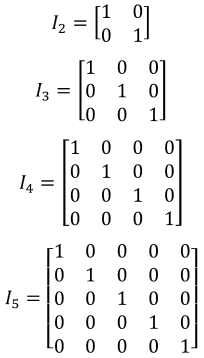# Identity matrix#### Everything You Need in One Place

Homework problems? Exam preparation? Trying to grasp a concept or just brushing up the basics? Our extensive help & practice library have got you covered.#### Learn and Practice With Ease

Our proven video lessons ease you through problems quickly, and you get tonnes of friendly practice on questions that trip students up on tests and finals.#### Instant and Unlimited Help

Our personalized learning platform enables you to instantly find the exact walkthrough to your specific type of question. Activate unlimited help now!

0/1
##### Intros
###### Lessons
1. The Identity Matrix
0/9
##### Examples
###### Lessons
1. Matrix Operation with the Identity Matrix
You are given that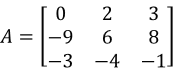and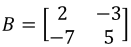. Perform the following matrix operations:
1. $I_3 \cdot A$
2. $2A+4I_3$
3. $-4B+2I_2$
4. $I_2 \cdot B$
5. $0 \cdot I_4$
2. Multiplicative Inverses
Are the following matrices multiplicative inverses of each other?

1.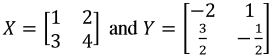2.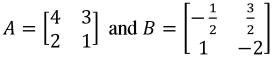3.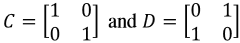4.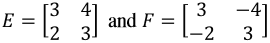###### Topic Notes
In this lesson, we will learn about identity matrices. Identity matrix is an n by n matrix which all entries diagonal from the top left to the bottom right are 1's, and the rest of the entries are 0. There are many types of identity matrices, as listed in the notes section. We will learn how to apply matrix operations with these such as adding, subtracting, and multiplying. Lastly, we will see that identities have a special property. If two matrices are multiplicative inverses, then multiplying them would give an identity matrix.

### Identity Matrix

Having learned about the zero matrix, it is time to study another type of matrix containing a constant specific set of values every time, is time for us to study the identity matrices.

### What is an identity matrix?

An identity matrix is a given square matrix of any order which contains on its main diagonal elements with value of one, while the rest of the matrix elements are equal to zero.

To explain part by part this definition, let us start by reminding you that a square matrix refers to a matrix containing the same amount of rows and columns. The order of a matrix comes from its dimensions, and its main diagonal refers to the array of elements inside the matrix which form an inclined line from the top left corner to the bottom right corner. Given the characteristics of an identity matrix, we can also conclude these type of matrices are also diagonal matrices.

A diagonal matrix is that in which all of its element entries are equal to zero, except for the elements found on its main diagonal. In this case, all of the non-zero entries in the matrix will have a value of one, and that happens to be one of the reasons why the identity matrix is sometimes called the unit matrix too.
For the case of matrix linear algebra notation, the identity matrix serves as the equivalent object to the unit in numerical algebra (other reason why is called the unit matrix). In other words, the identity matrix is the equivalent to the unit of one, but in this case it happens to be an algebraic object with dimensions and array organization which can be used in operations with other ordered number arrays (other matrices).
In the next section we will take a look into the properties of the identity matrix, and the unit matrix definition will make much more sense, especially in the case of matrix multiplications including an identity matrix (look at property number 3). Mathematically, the identity matrix is represented as:
Not to be confused with the identity of a matrix, notice the unit matrix has as many main diagonal elements as its order (denoted by the subindex n in the term found on the left hand side of the equation). Examples of identity matrices of different dimensions can be seen in the next equation:
Remember that the order of a matrix refers to the amount of rows and columns it contains, which are also called its dimensions mxn. Notice that since an identity matrix is a square matrix, it contains the same amount of rows and columns and so, its order can be referred simply as nxn, or as a one digit subindex as shown in equations 1 and 2, which we call "n" (given that both m and n dimensions coming from the typical matrix notation are equal for square matrices, only one of those letters is necessary as a subindex to describe the order of such matrices).
And so, in equation 2 we can easily see that I2 refers to an identity matrix with two rows and two columns, which at the same time has only two elements in its main diagonal; a notation of I3 corresponds to an identity matrix of order 3, or one containing three rows and three columns and 3 elements on its main diagonal; and the notation system continues that way for any subindex n.

### Identity matrix properties

1. An identity matrix is always an square matrix:
As seen in equations 1 and 2, the order of an identity matrix is always n, which refers to the dimensions nxn (meaning there is always the same amount of rows and columns in the matrix).
2. An identity matrix is capable of multiplying any matrix with any order (dimensions) as long as it follows the next rules:
1. If in the multiplication, the identity matrix is the first factor, then the identity matrix must have dimensions with as many columns as the matrix it is multiplying has rows.
2. If the identity matrix is the second factor in the multiplication, the identity matrix must have the same amount of rows as the matrix that is multiplying it has column
These two rules come from the conditions of matrix multiplication.
3. As a result of the first two rules, If an identity matrix is multiplying a square matrix of the same dimensions, the result will also be a square matrix which will be the same as the non-unit matrix of the multiplication, no matter the order in which the matrices are being multiplied with one another.
3. Following the two equations from the second property explained above, from all the identity matrix properties, the main one can be observed clearly in equations 3 and 4: Whenever an identity matrix multiplies another matrix, and the multiplication can be solved (following the rules for matrix multiplication), the result is equal to the non-unit matrix involved. Mathematically speaking:
If you think about it, this is the equivalent to multiplying a regular real number by the unit (by one). Any number multiplied by one results in the same original number. The same goes for a matrix multiplied by an identity matrix, the result is always the same original non-identity (non-unit) matrix, and thus, as explained before, the identity matrix gets the nickname of "unit matrix".
4. Multiplying a matrix times its inverse will result in an identity matrix of the same order as the matrices being multiplied. Mathematically speaking:
The matrices involved in such multiplication are called multiplicative inverses of each other. We will leave the explanation about inverse matrices for later lessons, starting with the topic of the inverse of a 2x2 matrix. For now, it is just important that you know this is one of the properties of identity matrix that we can use to solve matrix equations.
5. The determinant of the identity matrix In is always 1, and its trace is equal to n. Although we have not seen what a determinant is so far, for the moment is important to know that the reason a determinant of any identity matrix is equal to one is because the diagonal of these matrices contains only ones and the rest of the elements inside these matrices are zeros. We will introduce the concept of determinant in a later lesson called the determinant of a 2x2 matrix, where the identity matrix determinant will be explained in more detail.
On the other hand, the trace is much simpler to explain. By now you should know that the trace of a matrix refers to the addition of the elements found in its main diagonal. Since an identity matrix has diagonal elements all equal to one, when adding them all in order to obtain the trace, you will always have as many elements as the order of the identity matrix, which is n. Take the next identity matrix as an example:
Equation 7 shows an identity matrix 3x3, thus n=3 for this matrix. In that way, the trace is the addition of the elements of its diagonal, which is three elements of value 1 added with one another, and so, the trace is equal to 3. Therefore, the trace of an identity matrix is equal to n.
6. For our last property we start with a question: is the identity matrix invertible? The answer is yes. We will explain more on this topic through our lesson on about the 2x2 invertible matrix, for now just remember: The inverse of the identity matrix is itself.
We can prove the inverse of identity matrix is itself by multiplying them together. Remember from our fourth property that multiplying a matrix by its inverse produces the identity matrix, and so, we have computed the corresponding multiplication for this case (shown in equation 8) and proved that the inverse of a 2x2 identity matrix is itself.

### Identity matrix example problems

Making use of the properties learnt in the past section and the identity matrix definition given at the beginning of this lesson, solve the next example problems:

Example 1

Given the matrices below:
Perform the following matrix operations:
1. $I_{3} \cdot A$
On this case we have an example of the third property of the identity matrix: A multiplication involving an identity matrix and any other matrix, if defined due the rules of matrix multiplication, the result is the non-unit matrix (for this case, matrix A). And so, this multiplication goes as follows:
2. $2A + 4I_{3}$
For this matrix operation we are computing two cases of scalar multiplication, the second one including a 3x3 identity matrix. Solving such operation goes as:
3. $-4B + 2I_{2}$
Once more, this operation consists on using an identity matrix for addition of two scalar multiplications, where the second multiplication includes an identity matrix 2x2. We solve them as shown below:
4. $I_{2} \cdot B$
By the third property, we know the result of this multiplication is matrix B: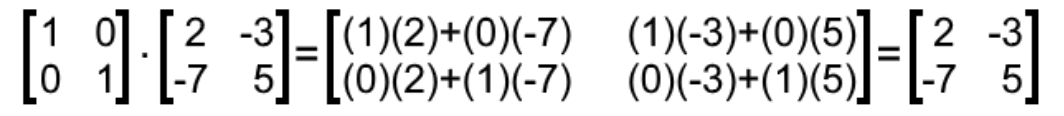Equation 13: Multiplying a 2x2 identity matrix to another matrix of same order
5. $0 \cdot I_{4}$
On this case, we have a scalar multiplication of an identity matrix with the number zero, thus, the result must be a zero matrix as shown below: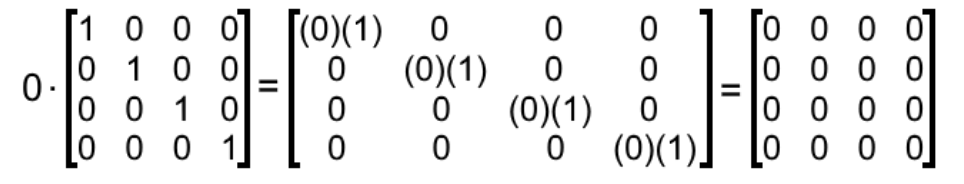Equation 14: Scalar multiplication of an identity matrix producing a zero matrix

Example 2

Show if the following matrices are multiplicative inverses of each other:
1. Having matrices X and Y as shown below:
We perform the matrix multiplication of X $\cdot$ Y:
Given that the result is the identity matrix, we conclude that X and Y are multiplicative inverses of each other.
2. Having matrices A and B as shown below:
We perform the matrix multiplication of A $\cdot$ B:
Thus, A and B are multiplicative inverses of each other.
3. Having matrices C and D as shown below:
We perform the matrix multiplication of C $\cdot$ D:
Although very similar, the resulting matrix from the operation above is not an identity matrix. Therefore, this operation shows that C and D are NOT multiplicative inverses of each other.
4. Having matrices E and F as shown below:
We perform the matrix multiplication of E $\cdot$ F:
An identity matrix is an $n$ by $n$ matrix (written as $I_n$) where all the entries that is diagonal from the top left to the bottom right are all 1's, and the rest of the entries are 0. For example,